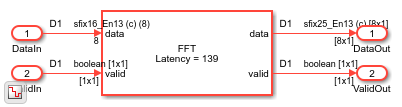# Automatic Delay Matching for the Latency of FFT Block

This example shows how to programmatically obtain the latency of an FFT block in a model. You can use the latency value for delay matching of parallel data paths.

Be cautious with delay matching for large signals or long latencies, since it adds memory to your hardware implementation. Alternatively, if the signal does not change within a frame, use the valid or frame control signals to align the signal with the output of the FFT block.

Open a model that contains an FFT or an IFFT block, such as the model from the Implement FFT Algorithm for FPGA example.

```modelname = 'FFTHDLOptimizedExample_Streaming'; load_system(modelname); set_param(modelname,'SimulationCommand','Update'); open_system([modelname '/FFT Streaming']); ```This example includes a `ffthdlLatency` function that calculates the latency of the block for its current parameters. Call the function with the block pointer and input vector size. You can use the Simulink path to the block, or select the block in the model to obtain a pointer, `gcb`. In this model, the input signal is a vector of 8 samples.

```latency = ffthdlLatency([modelname '/FFT Streaming/FFT'],8) ```
```latency = 139 ```

The function copies the parameters from the block pointer and creates a System object™ with the same settings as the block. Then it calls the `getLatency` function on the object. See `getLatency`.

```function lat = ffthdlLatency(block, vectorsize) % default vector size = 1 if nargin == 1 vectorsize = 1; end fftlen = evalin('base',get_param(block, 'FFTLength')); arch = get_param(block, 'Architecture'); bri = strcmpi(get_param(block, 'BitReversedInput'), 'on'); bro = strcmpi(get_param(block, 'BitReversedOutput'), 'on'); fftobj = dsphdl.FFT('FFTLength',fftlen, ... 'Architecture', arch, ... 'BitReversedInput', bri, ... 'BitReversedOutput', bro); lat = getLatency(fftobj, fftlen, vectorsize); end ```